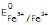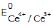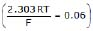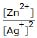Courses

# Previous Year Questions - Electrochemistry (IIT-JEE Advanced) JEE Notes | EduRev

## JEE : Previous Year Questions - Electrochemistry (IIT-JEE Advanced) JEE Notes | EduRev

The document Previous Year Questions - Electrochemistry (IIT-JEE Advanced) JEE Notes | EduRev is a part of JEE category.
All you need of JEE at this link: JEE

1. Calculate the equilibrium constant for the reaction, 2Fe3+ + 3I- 2Fe2+ + I3-. The standard reduction potentials in acidic conditions are 0.77 and 0.54 V respectively for Fe3+/Fe2+ and I3- /I- couples.

2. Find the solubility product of a saturated solution of Ag2CrO4 in water at 298 K if the emf of the cell Ag|Ag+ (satd. Ag2CrO4 soln.)||Ag+(0.1 M)|Ag is 0.164 V at 298 K. [JEE-1998]

5. Copper sulphate solution (250 mL) was electrolysed using a platinum anode and a copper cathode. A constant current of 2 mA was passed for 16 mintue. It was found that after electrolysis, the absorbance (concentration) of the solution was reduced to 50% of its original value. Calculate the concentration of copper sulphate in the solution to begin with. [JEE-2000]

6. The following electrochemical cell has been set up

Pt(I)|Fe3+, Fe2+(a=1)||Ce4+, Ce3+(a=1)|Pt(II)= 0.77 V and= 1.61 V

If an ammetter is connected between the two platinum electrodes. Predict the direction of flow of current. Will the current increase or decrease with time? [JEE-2000

10. The standard potential of the following cell is 0.23 V at 15ºC & 0.21 V at 35ºC

Pt|H2(g)|HCl(aq)|AgCl(s)|Ag(s) [JEE-2001]

(i) Write the cell reaction.

(ii) Calculate DHº, DSº for the cell reaction by assuming that these quantities remain unchanged in the range 15ºC to 35ºC.

(iii) Calculate the solubility of AgCl in water at 25ºC. Given standard reduction potential of the Ag+/Ag couple is 0.80 V at 25ºC

13. Two students use same stock solution of ZnSO4 and a solution of CuSO4. The e.m.f of one cell is 0.03 V higher than the other. The conc. of CuSO4 in the cell with higher e.m.f value is 0.5 M. Find out the conc. of CuSO4 in the other cell. [JEE-2003]

17. (a) Calculate DGf0 of the following reaction

Ag+(aq) + Cl-(aq) ® AgCl(s)

given DfGº (AgCl) = - 109 kJ/mole,

DfGº (Cl-) = - 129 kJ/mole,

DfGº (Ag+) = 77 kJ/mole

Represent the above reaction in form of a cell

Calculate Eº of the cell. Find log10KSP of AgCl

(b) 6.539 × 10-2 g of metallic Zn (amu = 65.39) was added to 100 ml of saturated solution of AgCl.

Calculate log10, given that

Ag+ + e- ® Ag Eº = 0.80 V ; Zn2+ + 2e- ® Zn Eº = - 0.76 V

Also find how many moles of Ag will be formed? [JEE-2005]

21. We have taken a saturated solution of AgBr. Ksp of AgBr is 12 × 10-14. If 10-7 mole of AgNO3 are added to 1 litre of this solution find conductivity (specific conductance) of this solution in terms of 10-7 S m-1mole-1.

Offer running on EduRev: Apply code STAYHOME200 to get INR 200 off on our premium plan EduRev Infinity!

,

,

,

,

,

,

,

,

,

,

,

,

,

,

,

,

,

,

,

,

,

;